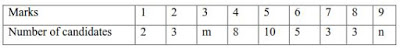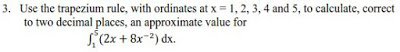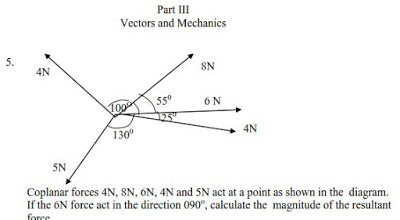Members :: 19114

### WAEC Past Questions And Answers On Further Mathematics 2021

⭐⭐⭐⭐⭐ - Information on WAEC Past Questions And Answers On Further Mathematics. Other topics of interest include: ACCA Past Papers F8, ACCA Past Exam Papers, ACCA F9 Past Papers, ACCA F3 Past Papers, ACCA Past Papers P7. Use the search box above to search for more.

Notice:

FURTHER MATHEMATICS/MATHEMATICS (ELECTIVE) EXAMINATION SCHEME

There will be two papers, Papers 1 and 2, both of which must be taken. PAPER 1: will consist of forty multiple-choice objective questions, covering the entire syllabus. Candidates will be required to answer all questions in 1 hours for 40 marks. The questions will be drawn from the sections of the syllabus as follows:

Pure Mathematics – 30 questions Statistics and probability – 4 questions Vectors and Mechanics – 6 questions

PAPER 2: will consist of two sections, Sections A and B, to be answered in 2 hours for 100 marks. Section A will consist of eight compulsory questions that are elementary in type for 48 marks.

The questions shall be distributed as follows: Pure Mathematics – 4 questions Statistics and Probability – 2 questions Vectors and Mechanics – 2 questions Section B will consist of seven questions of greater length and difficulty put into three parts: Parts I, II and III as follows: Part I: Pure Mathematics – 3 questions Part II: Statistics and Probability – 2 questions Part III: Vectors and Mechanics – 2 questions Candidates will be required to answer four questions with at least one from each part for 52 marks.

SAMPLE QUESTIONS

PAPER 1 (OBJECTIVES) 1. Find the equation of the line joining points (8, 1) and (-3, 4). A. 3x – 11y – 35 = 0 B. 3x – 11y + 35 = 0 C. 3x + 11y – 35 = 0 D. 3x + 11y + 35 = 0 2. The sum of the first and sixth terms of an Arithmetic progression (A.P.) is 21. If the first term is 3, find the eighth term. A. 24 B. 27 C. 30 D. 33 3. If and are the roots of the equation 2×2 – 5x + m = 0, where m is a constant, find ( 2 + 2) in terms of m. A. + 2m B. + m C. – 2m D. – m 4. Given that y =cos2x, find A. -sin2x B. –cos x sin x C. -2cosx sinx D. -2sin2x 5. The position vectors of points P, Q and R are p = 4j, q = (4i + 10j) and r = (ki + 8j) respectively, where k is a constant. If ∠PQR = 90o, find the value of k. A. 7 B. 1 C. -1 D. -7

PAPER 2 (ESSAY) SECTION A 1. Given that * is a binary operation defined on R, the set of real numbers by x*y = x2/x+y , where x, y R. (a) evaluate (2 * 3) * 5. (b) If (x + 1) * (x + 2) = , find the value of x. 2. The marks scored by forty candidates in an examination are shown in the table.If the mean of the distribution is 4.725, find the values of m and n.

SECTION B Part I (Pure Mathematics)Part II (Statistics and Probability) 4. The deviations from 10 of a given set of numbers are 2, 1, 0, -4, -5, – 1, – 2 and – 7. Find the: (i) mean; (ii) median; (iii) standard deviation of the numbers.View Trending Twitter Hashtags & Topics In Ghana Live!

#### WAEC E-Learning Portal Tool

Use CTRL + P To Print From The Page

The syllabus application works best on a tablet or desktop machine. In case you cannot view, try using a full web browser.

### Print Out CSSPS Placement forms from the Internet

WAEC Ghana Telephone Contact Numbers Directory

WAEC Ghana & Nigeria Head Office Website

WAEC Ghana MasterCard / VISA Card E-payment Online Service

Ministries
Accra
Contact
T 030 2662977
T 030 2223620
T 030 2665534

visitors online

### Join Chat

 Login to chat with other membersRooms available: Members logged in (19) :
Page Last Updated On Thursday, 23 September 2021.